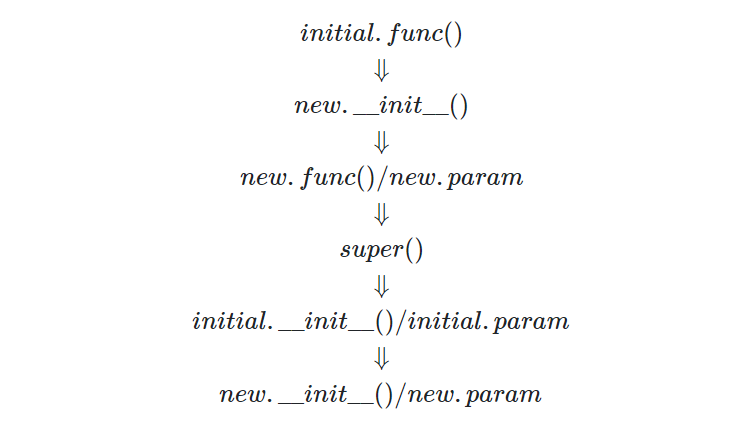# python中的super是什么？本文通过一个python的实际案例的设计，来讲解python面向对象的技术——类的继承中必用的super函数的逻辑。其实我们可以把python中类的继承理解成这样的一个过程：当我们在括号中明确了父类时，其实已经引用了父类的成员函数，但是并没有执行父类的初始化函数。在执行子类的初始化函数的同时，会检查是否重载了父类的成员函数，如果重载则会直接覆盖。而只有在执行了super之后，才相当于执行了父类的初始化函数，此时才可以访问父类的成员变量。

# 技术背景

python中的super，名为超类，可以简单的理解为执行父类的__init__函数。由于在python中不论是一对一的继承，还是一子类继承多个父类，都会涉及到执行的先后顺序的问题。那么本文就着重看下super的具体作用。

# 案例测试

``````# 定义父类
class initial(object):
def __init__(self):
print ('This print is from initial object')
# 定义父类参数
self.param = 3

# 定义父类函数
def func(self):
return 1

# 定义子类
class new(initial):
def __init__(self):
print ('This print is from new object')
# 打印子类函数值
print (self.func())
# 执行父类初始化函数
super(new, self).__init__()
# 打印父类参数值
print(self.param)
self.param = 4

# 定义子类函数
def func(self):
return 2

if __name__ == '__main__':
new()
``````

``````This print is from new object
2
This print is from initial object
3
``````

## 结果分析

``````class initial(object):
def __init__(self):
print ('This print is from initial object')
self.param = 3
def func(self):
return 1

class new(initial):
def __init__(self):
print ('This print is from new object')
print (self.func())
super(new, self).__init__()
print(self.param)
self.param = 4

if __name__ == '__main__':
new()
``````

``````This print is from new object
1
This print is from initial object
3
``````

\[initial.func()\\ \Downarrow\\ new.\_\_init\_\_()\\ \Downarrow\\ new.func()/new.param\\ \Downarrow\\ super()\\ \Downarrow\\ initial.\_\_init\_\_()/initial.param\\ \Downarrow\\ new.\_\_init\_\_()/new.param \]